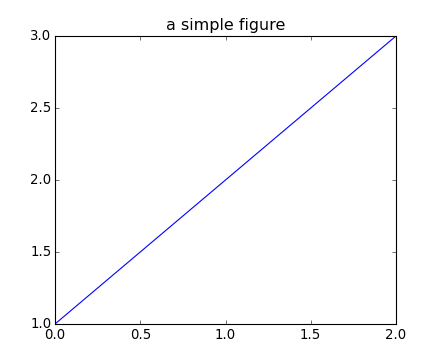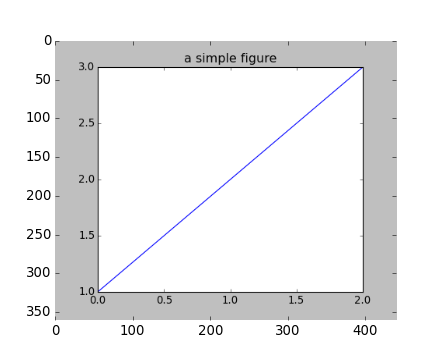#### Previous topic

pylab_examples example code: accented_text.py

#### Next topic

pylab_examples example code: alignment_test.py

# pylab_examples example code: agg_buffer_to_array.py¶(png, hires.png, pdf)(png, hires.png, pdf)

```import matplotlib.pyplot as plt
import numpy as np

# make an agg figure
fig, ax = plt.subplots()
ax.plot([1,2,3])
ax.set_title('a simple figure')
fig.canvas.draw()

# grab the pixel buffer and dump it into a numpy array
buf = fig.canvas.buffer_rgba()
l, b, w, h = fig.bbox.bounds
# The array needs to be copied, because the underlying buffer
# may be reallocated when the window is resized.
X = np.frombuffer(buf, np.uint8).copy()
X.shape = h,w,4

# now display the array X as an Axes in a new figure
fig2 = plt.figure()